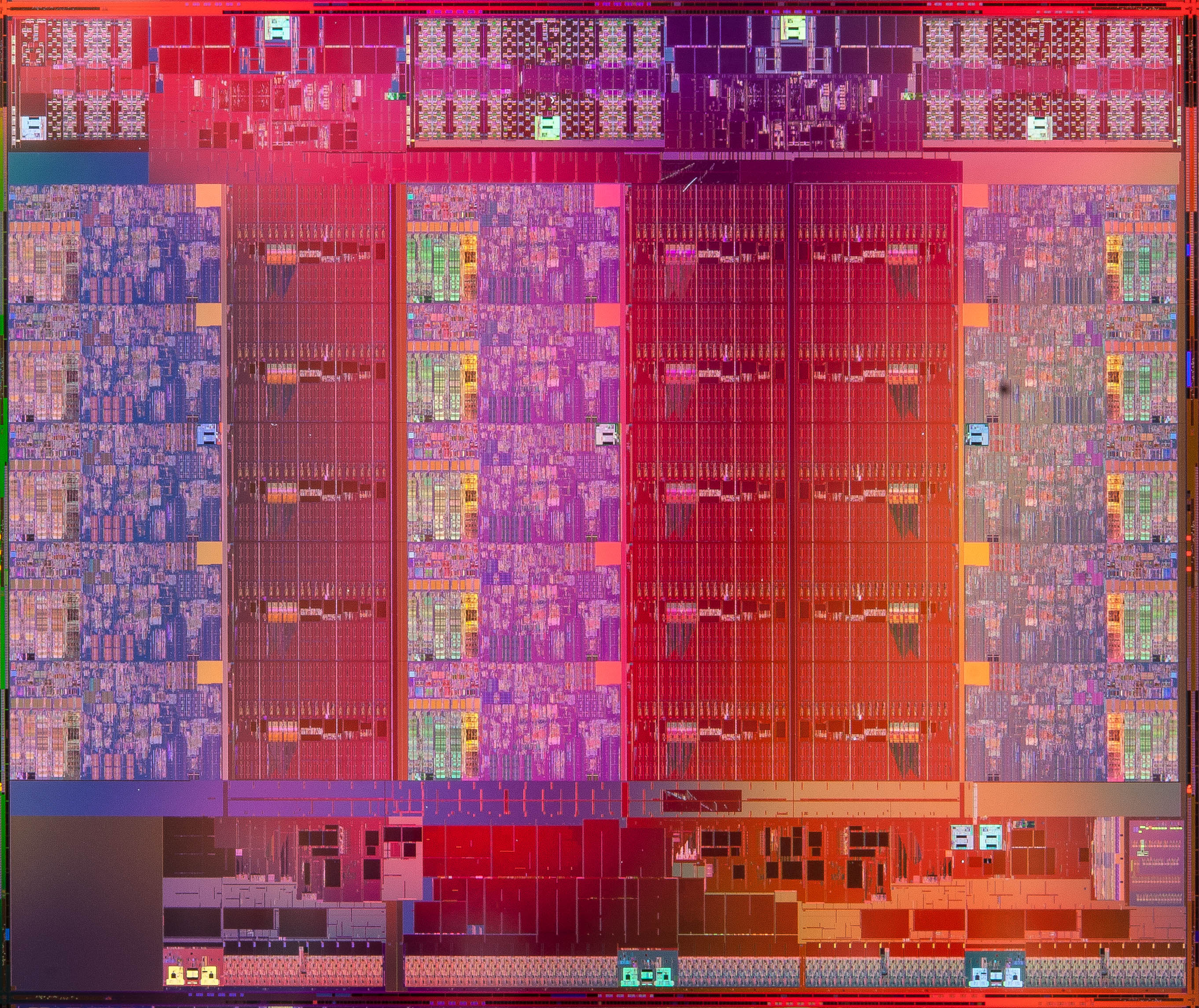# Module I: The mystery of the computer(An Intel Xeon E7 v2 die, 15 cores, 4.3 billion 22nm transistors. Intel Newsroom Photo Gallery.)

Having learnt the fundamentals of the art of computer programming, an aspiring computer scientist ought to pause and reflect at the capabilities of this marvellous artefact that obediently executes our programs.

Copy and paste the following fragment of code into a console:

def test(m: Long) = {
var i = 1L
var s = 0L
while(i <= m) {
s = s + i
i = i + 1
}
s
}

val NANOS_PER_SEC   = 1e9
val test_start_time = System.nanoTime
test(4000000000L)
val test_end_time   = System.nanoTime
val test_duration   = test_end_time - test_start_time
println("the test took %.2f seconds".format(test_duration/NANOS_PER_SEC))


We observe the following:

the test took 2.02 seconds


(This on an 2020 Dell laptop running an Intel Core i5-8365U CPU @ 1.60GHz and 16 GiB of main memory.)

In just over two seconds, the machine has evaluated the sum of the first four billion positive integers. This means that the machine must make, roughly, two billion loop iterations each second. That is, just under two loop iteration every nanosecond.

(The speed of light in vacuum is roughly $$3.0\times 10^8$$ meters per second. In one nanosecond, that is, $$1.0\times 10^{-9}$$ seconds - roughly two loop iterations - light travels about 30 centimetres.)

It thus appears to be quite a powerful artefact that we have at our command.

But it is only a machine. How does it operate? By what principles?

How are such machines designed and built?

How does the machine accept our programs and do what we instruct it to do by means of, for example, the Scala programming language?

What is computation?

This is what we will set out to understand in Module I, while learning further aspects of the art of computer programming.